When to Use SUM() vs SUMX() in DAX ? Excelerator BI

# When to Use SUM() vs SUMX() in DAX

Level: Beginners/Intermediate (originally posted 2015, updated 2017, 2018, 2022).

Before moving on to SUM() vs SUMX(), there are 2 important concepts about how Power BI and Power Pivot for Excel work that you must understand.

## Things You Should Know Before I Cover SUM() vs SUMX()

### Filter Behaviour

Before getting into SUM() vs SUMX(), it’s important to know that when you write a formula in DAX, the result of the formula depends on which filters have been applied in the report.  DAX is not the same as Excel. In Excel you can write slightly different formulas in each and every cell of the report and each formula can itself point to different cells creating different results.  Every cell is stand alone and unique.  That is not how it works in DAX.  In DAX you write a single formula such as SUM(Sales[Sales Amount]) and then use filters in the report to modify the results returned from the formula.

Filters can come from:

1. The visuals in your workbook. A Pivot Table if you are using Power Pivot for Excel, or anywhere on the report canvas if you are using Power BI.  The visuals in your report create the Initial Filters that impact your formulas.
2. The use of a CALCULATE() function.  CALCULATE() is the only* function that can change the Initial Filter behaviour of your report.  Of course there may or may not be a CALCULATE() function in your DAX formula (or a precedent formula).  If there is a CALCULATE() function, it can add to, remove from, or modify the initial filter behaviour of the formula.

* Note: Filters can also be modified by an implicit CALCULATE() in a measure, CALCULATETABLE() and also functions that are simplified versions of CALCULATE(), such as TOTALYTD()

Filters always get applied first, then the evaluation is completed.  Filters first, evaluate second. The technical term for this filter behaviour is “Filter Context”, but I prefer to use the term “Filter Behaviour” as it is less intimidating for most people.

Filter behaviour is important because it will affect the results you get from your formulas.  Let’s briefly look at how the initial filters work in Excel and Power BI.

### Power Pivot for Excel

In the following Pivot Table example, the highlighted cell has an initial filter of Products[Category] = “Bikes” coming from the rows of the pivot table (shown as 1).  Initial filters can also come from Filters, Columns and Slicers in a pivot table.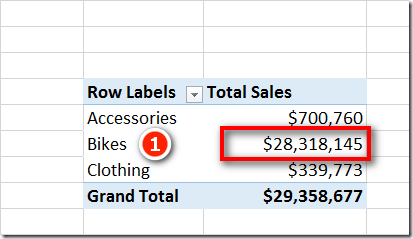### Power BI Desktop

Power BI Desktop is similar to Excel, but initial filters can come from almost anywhere in the report.  The highlighted cell below is filtered by Products[Category]=”Bikes” (shown as 1) just like in Excel above, but there is also a cross filter coming from Territory[Country]=”Australia” (shown as 2).  Both of these filters are part of the initial filters.  Filters can also come from Columns, Slicers, and the filter section on the right hand side of the Power BI report window.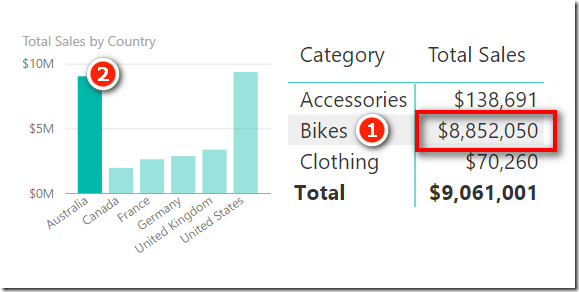Now onto a new topic.

## Row By Row Evaluation

A second important topic for you to understand is ‘row by row evaluation’.  Not every DAX formula is capable of doing calculations row by row.  Sometimes DAX has the ability to be evaluated row by row (such as in a calculated column) and sometimes it cannot (as in a regular measure). [As an aside, this is single handily the most difficult concept to learn in the DAX language and it is covered in my various Power BI courses.] You can write a single formula in a calculated column in the Sales table, such as Sales[Qty] * Sales[Price Per Unit] and this formula is evaluated one row at a time down the entire column.  If you were to write this exact same formula as a measure, you would get an error.

The technical term for this behaviour is “Row Context”, but I prefer to use the term “Row by Row Evaluation” as it is less intimidating for most people.### SUM() vs SUMX() in a nutshell

• SUM() operates over a single column and has no awareness of individual rows in the column (no row by row evaluation).
• SUMX() can operate on multiple columns in a table and can complete row by row evaluation in those columns.

Both functions can end up giving you the same result (maybe, maybe not), but they come up with the answer in a very different way (*more on that later).  Both SUM() and SUMX() often give the same results inside the rows of a matrix or visual, but often give different results in the sub totals and totals section of a visual.

### The SUM() Function

Syntax: = SUM(<Column Name>)

Example: Total Sales = SUM(Sales[ExtendedAmount])

The SUM() function operates over a single column of data to aggregate all the data in that single column with the current filters applied – filter first, evaluate second.

### The SUMX() Function

Syntax: = SUMX(<Table>, <expression> )

Example: Total Sales SUMX = SUMX(Sales, Sales[Qty] * Sales[Price Per Unit])

SUMX() will iterate through a table specified in the first parameter, one row at a time, and complete a calculation specified in the second parameter, eg Quantity x Price Per Unit as shown in the example above with the current filters applied (i.e. still filter first, evaluate second).  Once it has done this for every row in the specified table (after the current filters are applied), it then adds up the total of all of the row by row calculations to get the total.   It is this total that is returned as the result.

## SUM() vs SUMX(): Which One Should I Use?

Whether to use SUM or SUMX  in DAX really depends on your personal preference and the structure of your data.  Let’s look at a couple of examples.

1. Quantity and Price Per Unit
2. Extended Amount

### 1. Quantity and Price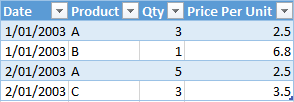If your Sales table contains a column for Quantity and another column for “Price Per Unit” (as shown above), then you will necessarily need to multiply (one row at a time) the Quantity by the “price per unit” in order to get Total Sales.  It is no good adding up the total quantity SUM(Quantity) and multiplying it by the average price AVERAGE(Price Per Unit) as this will give the wrong answer.

If your data is structured in this way (like the image above), then you simply must* use SUMX()  – this is what the iterator functions were designed to do.  Here is what the formula would look like.

Total Sales 1 =SUMX(Sales,Sales[Qty] * Sales[Price Per Unit])

You can always spot an Iterator function as it always has a table as the first input parameter.  This is the table that is iterated over by the function.

*Note:  I say must, but actually this is where many (most) business people tend to make  a mistake.  To solve this problem, rather than using SUMX() as prescribed above, many business people tend to gravitate towards a calculated column to solve the problem.  A calculated column solves the problem in the same way as SUMX(), but with one big difference – it permanently stores the row by row results in in a new column in the table.  This is generally bad and you should avoid this.  I recommend you read my article Measures vs Calculated Columns for a more in depth coverage of this topic.

### 2. Extended Amount

If your data contains a single column with the Extended Total Sales for that line item (ie it doesn’t have quantity and price per unit), then you can use either SUM() or SUMX() to add up the values.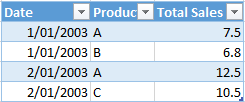Total Sales 2 =SUM(Sales[Total Sales])

Total Sales 2 alternate = SUMX(Sales, Sales[Total Sales])

### Syntax Sugar

Despite what your intuition may tell you, this alternate formula using SUMX() is identical in performance and efficiency to the SUM() version.  In fact, they are technically the same formula.  SUM(Table[Column]) is just syntax sugar for SUMX(Table,Table[Column]).  Syntax sugar refers to a simplified way of writing a formula that is easier to understand and easier to write, but under the hood they are identical. Note, the simplified version using SUM can only operate over a single column. If you want to operate over multiple columns, you must use the full SUMX syntax.

### 3. Totals Don’t Add Up

There is another use case when you simply must use SUMX() that is less obvious. When you encounter the problem where the totals don’t add up as you need/expect, you will need to use an iterator like SUMX to get the correct total.  I have created a small table of sample data to explain.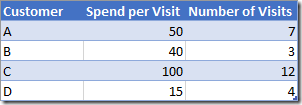The table above shows 4 customers with the average amount of money they spend each time they have shopped as well as the number of times they have been shopping. If I load this data into Power BI and then try to use aggregator functions to find the average spend across all customers as well as the total amount spent, I get the wrong answers in the total row (as shown below).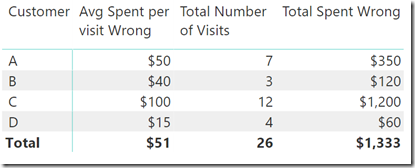Here are the measures from above.

Total Number of Visits = SUM(VisitData[Number of Visits]) – the total is correct for this formula.

Avg Spent per visit Wrong= AVERAGE(VisitData[Spend per Visit]) – the total is wrong here.

Total Spent Wrong = [Avg Spent per visit Wrong] * [Total Number of Visits] – the total is wrong here too.

The first measure [Total Number of Visits] is correct because the data is additive, but the other 2 measures give the wrong result on the total row despite giving the correct result on the rows within the table. This is a classic situation where you can’t perform multiplication on the averages at the grand total level. Given the sample data that I started with, the only way to calculate the correct answer is to complete a row by row evaluation for each customer in the table as shown below.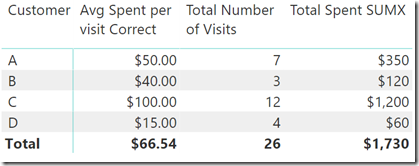In this second table above I have written a SUMX() to create the Total Spent SUMX (row by row) first. Only then do I calculate the average spend per visit as the final formula.

Total Number of Visits = SUM(VisitData[Number of Visits])
Total Spent SUMX = SUMX(VisitData,VisitData[Spend per Visit] * VisitData[Number of Visits])
Avg Spent per visit Correct = DIVIDE([Total Spent SUMX] , [Total Number of Visits])

In this second case, SUMX is working through the table of data one row at a time and is correctly calculating the result, even for the total row at the bottom of the table.

## Final Thoughts on SUM() vs SUMX()

As always, there are exceptions to the rules.  Everyone’s data is different, so test out the techniques shown when comparing SUM() vs SUMX() on your own data and see what gives you the best results.  If your data models are small and fast then it probably doesn’t matter.  If your data models start to get large and slow, then it is time to investigate the best options to try to maximise performance.

If you found it easy to learn from this article, then you may like to consider completing some more structured learning from me.  You can learn more from me in different ways, including:

These invaluable tools are sure to assist you and all have the same “easy to learn and easy to understand” approach.

### 52 thoughts on “When to Use SUM() vs SUMX() in DAX”

1.Great explanation

2.Fantastic explanation ! Thanks a lot for posting this.

3.Thanks Matt, very helpful to understand these two functions.

Carlos

4.You are very good. I respected👍🏻

5.This is awesome

6.Matt, this is very well explained, thanks a lot.

7.Great article with the concept explained in a easy to understand language.

8.Great article thanks

9.Hi Matt;
I’m new to PowerBI, and working through it. I have a cash flow table with columns representing each month and rows representing projects. I need to run a cumulative total over time, totalling columns rather than rows:
Project Jan Feb Mar
Proj 1 2 3 4
Proj 2 3 4 5
Total 5 7 9
Cumulative: 5 12 21
I have seen various example for running totals down a column, I am looking to accumulate column totals in a row. Can you help me out?

1.I suggest you ask for help at community.powerbi.com

10.Matt,
Great articale. I’m trying to solve performance issues on the following calc, which return good result but very bad respond time.
How do you suggest solving it?
Measure = CALCULATE(
SUMX(
GROUPBY(Sales,
[Product_Id],[Month_of_Sale],
“MaxSale”,
MAXX(CURRENTGROUP(),[Total_Sale])
),
[MaxSale])
,VALUES(Main_Slicer_Tbl[Value])
,CALCULATETABLE(ALLSELECTED(Sub_Slicer_Tbl))
)
Result should looks like:
MainSlicerVal SubSlicerVal Measure
Accessories #A123 1000
Bikes #B456 1400
Clothing #CB332 3000
Clothing #CA334 3000

1.You should ask your question in a forum. Feel free to then post a link to the post and I will look if I have time.

11.That’s great Tom. There is definitely value in repetition until you get it. In my experience, most people need a few goes to absorb the harder concepts.

12.Matt,
I’m sure I have read this a couple times before but now I am really getting it. Don’t know if I’m getting smarter or your revised article is that much better but thanks either way because if I am getting smarter it’s because of your articles.

13.Simple but great explenations. Thank you so much!

14.Excellent Matt. As always you have elucidated the concepts very effectively. The key underlying mechanics of how DAX works is essential and you have articulated well on this somewhat complex subject that can be digested easily.
Kudos

15.Great article. I’m slightly embarrassed how long I’ve been using Dax and avoided interators… but when teaching Dax to others, it’s become a nice example of the importance of writing a measure carefully… and ensuring correct totals all the way through to the grand total. Thanks!

16.Thanks Diogo. You have inspired me to do a refresh on this article to update it with my latest teaching techniques.

17.I really like how you are able to break such complex subjects into simple explanations.
Thank you very much for you work!

18.Thanks for sharing the Article !!
Little confused why the same calculation “Total Sales 1 =SUMX(Sales,Sales[Qty] * Sales[Price Per Unit])” will give different result if it Created as New Column or Created as Measure in Quantity and Price Table

1.It will give the same result. Did I suggest otherwise?

19.Your tip on replacing the if-statement with a Sumx helped a lot! First the pivottable would crash, afterwards it refreshed in just a few seconds!

20.Thank you very much for writing this piece of art. Awesome work!!!

21.Great article. Really interesting re performance differences of unique vs non-unique values. I use COUNTA() a lot and shall now review which columns I am using to count.

22.Bruce Montemayor

It might just be me being thick, but I’m not following why the example is using an average instead of a sum. SUM() vs. SUMX() is something I’ve been pondering for some time. I seem to get the same answer (If memory serves) whether I’m using:

SUM(Financials[Obligations]) – SUM(Financials[Expended]) or

SUMX(Financials,[Obligations]-[Expended])

In this situation, which is the one to use?

1.In your example, both columns are additive so it makes no difference. Identical performance and results. But if one column is quantity and another is price per unit, then you must use sumx.

2.@Bruce
SUM(Financials[Obligations]) is just short had for SUMX(Financials, [Obligations])
So my guess would be
SUMX(Financials,[Obligations]-[Expended]) would be faster

3.I have a production database with 39 million rows. I just tested three things
1. SUM(column1) + SUM(column2)
2. SUMX(table,column1) + SUMX(table,column2)
3. SUMX(table,column1 + column2)

1 and 2 delivered identical results with identical query plans averaging around 35 ms
3 had a slightly different query plan and consistently took twice as long at over 70ms. But given the difference is only 35 ms, I guess it doesn’t really matter – use which ever you want.

This is my data and it may be different on other data

1.Bruce Montemayor

Thanks All! Good info to know. Honestly, how impressive is DAX if we are pondering 35 ms over 39 million rows… Hey Microsoft, Good on ya! (I’m not an Aussie but like the lingo.) Thanks again!

2.@Matt – This is very interesting and strange- I want to try this on my Data as well
I just cant understand why this SUMX(table,column 1 + column 2) should be slower than
SUMX(table, Column 1) + SUMX(table, Column 2)
Time to shine the “Help Italians” light

3.I don’t profess to be speaking based on my knowledge, but I can conceive how this could be. If we assume that there is an overhead to add 2 columns together, and sumx(table, column1 + column2) is likely to have more addition events than sumx(table, column1) + sumx(table,column2), then there would be less overhead in the second one. That is my guess

1.@Matt – But what about the fact that you have 2 Iterators – Iterating over millions of rows.

2.@Matt – Thanks for the explanation. I am aware about data compression depending on the cardinality of the columns
So my guess is the speed between the two approaches would vary greatly depending on the cardinality of the two number columns – I guess in most real life scenarios this would be high if ex : Basic Amt + Tax Amt to arrive at net amount would be slow
Whereas lets assume Type + Rating = Some fictitious metric, where Type has 1, 2 and 3 and Rating has 1,2,3,4,5 would be fast

1.@Sam, that could be correct – I would be just guessing. I know the query plans between the 2 measures is different, so the engine is treating things a different way. Much of what The Italians do to optimise their DAX is done by reading the query plans and working out which is most efficient. Often this is a bit of trial, error and experience. There is a very good video where Alberto talks about this concept. I don’t remember which one, but this one looks good http://www.sqlbi.com/tv/optimizing-dax-queries/

4.@Sam, like I said I can only share what I think (I am not an expert). But I have attended Marco Russo’s Optimising DAX training and I have some understanding of what is happening. With this in mind, here are some comments.
A. Even though SUMX is an iterator, it doesn’t scan millions of rows. The engine is optimised and the data is compressed. The compression finds matches in the columns of data and stores it efficiently. Conceptually, if there were 2 unique values in a column but 4 million rows of data, the engine would only have to scan 2 rows, not 4 million (it is not exactly like that, but it should help conceptualise).
B. There are 2 different tasks for this example. 1) Add up the values in the columns and 2) add the columns together. Given the way that compression works and given the different overhead of the different tasks, there may be more overhead to add the columns together row wise than to add the columns first on their own and then add them together at the end.

23.Thanks for this, very well explained article. Have you got a book to buy? 🙂

1.Glad you like it. Yes I have a book – I us ether same approach in the book as you will find on my website. https://exceleratorbi.com.au/learn-to-write-dax/

24.Pawel Jaszczynski

very well explained, clear examples, thanks for sharing.

25.Claude Van Horn

I just got 2016 installed, and am giving Power Pivot another try. Mostly, I love it (and I love articles like this one that give great watch-points!) My question: I want to change column order in a dataset in Power Pivot.

I need to do that in the query, I understand that from the error message I get when I try to do it in Power Pivot. But when I change the column order in Power Query, some of the columns change to the new positions and some do not when I update the Power Pivot from the new query. I even tried deleting the table and bringing it in again from the new query after getting everything just as I want it.

I checked the Query Advanced Editor to make sure the Reorder Columns statement is correct, and it is. Query is in proper order, Power Pivot model is not. I realize it doesn’t make any difference in the resulting formulas or pivot tables, but if I look at the tables, the order is not what I want. Thanks for your help!

1.I agree it is strange, but also agree it doesn’t really matter. So you use the column name list at the top left of the data view grid? That is always alphanumeric order and will take you directly to the column you select.

26.To remember why you must use SUMX when multiplications between columns are involved (paragraphs 1 and 3 in your post), people should think of the order of operations in elementary school math (BODMAS / PEMDAS), where multiplication takes precedence over addition. SUMX is DAX’s way of enforcing proper order of operations.

27.This is one of the best articles on PowerPivot I have ever read, neatly incorporating every important concept in a clear and concise manner. Thanks a lot for helping us expanding our understanding of how PowerPivot really works and how to use it most efficiently!

1.Thanks Pascal, I appreciate you saying so.

28.1.Thanks Steve, yes I saw it 🙂

29.My understanding was that
SUM ( tblName[ColName] ) is just Syntax Sugar for SUMX (tblName, [ColName])
My understanding was SUM is also an Iterator – How can it be otherwise ?

1.Hi Sam, I certainly understand your comment. I had the same thought when starting to learn DAX.

SUM() definitely iterates through the values of whatever column is specified as the argument. That’s after all the only way to aggregate something. You need to have access to the individual values to do so.

SUM() is not an iterator because it does not own the right to set the DAX engine into row context mode (One of the 2 contexts a DAX expression is evaluated under even though either or both of them can be empty). Only an iterator can set the DAX engine into row context mode and SUMX() happens to be one.

Operating under Row context mode means that you can now refer to all the columns of a row instead of just one as is the case with SUM(). In other words it’s now legal syntax to reference more than one column. SUM(Sales[Qty] * Sales[Price Per Unit]) would not be legal syntax because of the aforementioned reasons, SUMX(Sales,Sales[Qty] * Sales[Price Per Unit]) is legal syntax because SUMX() is an iterator and therefore operates in row context mode. Both SUM() and SUMX() iterates through all the rows visible in the current filter context but SUMX() does so with more degrees of freedom so to speak.

You can say that SUM ( tblName[ColName] ) is just Syntax Sugar for SUMX (tblName, tblName [ColName]) because only one column is involved. The 2 expressions would give the same result in that case. Well as a matter of fact the correct syntax sugar for SUM ( tblName[ColName] ) would be SUMX (VALUES(tblName), tblName[ColName]). The difference is subtle and is only important if ‘ tblName’ is a dimension table and an unknown member exists on this table.

Best regards Jes.

1.@Oxenskiold – Thanks for your explanation. I understand what you mean by SUMX having access to any column of the table in the row that it is iterating.
However your last line got me confused
“SUM ( tblName[ColName] ) would be SUMX (VALUES(tblName), tblName[ColName]). The difference is subtle and is only important if ‘ tblName’ is a dimension table and an unknown member exists on this table”

Assuming tblName is a fact table what it the difference between
EVALUATE tblName and EVALUATE VALUES(tblName)
both seem to return a table with the same number of rows despite have duplicate rows.

1.Hi Sam,
“SUM ( tblName[ColName] ) would be SUMX (VALUES(tblName), tblName[ColName]). The difference is subtle and is only important if ‘ tblName’ is a dimension table and an unknown member exists on this table”

I almost regret writing this. Please standby for a few days.

1.@Oxenskiold – eagerly await your response !!

30.This is the best PowerPivot article I’ve ever read! Thanks for sharing!

1.Thanks so much Felipe

Scroll to Top